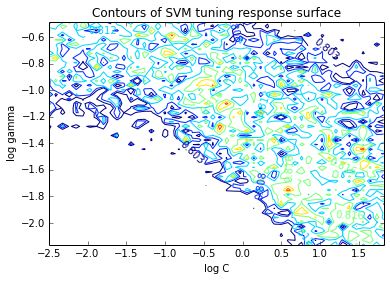Optunity is a library containing various optimizers for hyperparameter tuning. Hyperparameter tuning is a recurrent problem in many machine learning tasks, both supervised and unsupervised.This package provides several distinct approaches to solve such problems including some helpful facilities such as cross-validation and a plethora of score functions.

From an optimization point of view, the tuning problem can be considered as follows: the objective function is non-convex, non-smooth and typically expensive to evaluate. Tuning examples include optimizing regularization or kernel parameters.

The figure below shows an example response surface, in which we optimized the hyperparameters of an SVM with RBF kernel. This specific example is available at Optimization response surface.The Optunity library is implemented in Python and allows straightforward integration in other machine learning environments. Optunity is currently also supported in R, MATLAB, GNU Octave and Java through Jython.

If you have any problems, comments or suggestions you can get in touch with us at gitter:Optunity is free software, using a BSD-style license.

# Example¶

As a simple example of Optunity’s features, the code below demonstrates how to tune an SVM with RBF kernel using Optunity and scikit-learn. This involves optimizing the hyperparameters gamma and C:

import optunity
import optunity.metrics
import sklearn.svm

# score function: twice iterated 10-fold cross-validated accuracy
@optunity.cross_validated(x=data, y=labels, num_folds=10, num_iter=2)
def svm_auc(x_train, y_train, x_test, y_test, logC, logGamma):
model = sklearn.svm.SVC(C=10 ** logC, gamma=10 ** logGamma).fit(x_train, y_train)
decision_values = model.decision_function(x_test)
return optunity.metrics.roc_auc(y_test, decision_values)

# perform tuning
hps, _, _ = optunity.maximize(svm_auc, num_evals=200, logC=[-5, 2], logGamma=[-5, 1])

# train model on the full training set with tuned hyperparameters
optimal_model = sklearn.svm.SVC(C=10 ** hps['logC'], gamma=10 ** hps['logGamma']).fit(data, labels)


For more examples, please see our Examples.

# Quick setup¶

Issue the following commands to get started on Linux:

git clone https://github.com/claesenm/optunity.git
export PYTHONPATH=$PYTHONPATH:$(pwd)/optunity/


Afterwards, importing optunity should work in Python:

python -c 'import optunity'


For a proper installation, run the following:

python optunity/setup.py install


or, if you have pip:

pip install optunity


Installation may require superuser priviliges.

Optunity API

# Contributors¶

Optunity is developed at the STADIUS lab of the dept. of electrical engineering at KU Leuven (ESAT). The main contributors to Optunity are:

Marc Claesen

• Python package
• framework design & implementation
• solver implementation
• communication protocol design & implementation
• MATLAB wrapper
• Octave wrapper
• Python, MATLAB and Octave examples

Jaak Simm

• communication protocol design
• R wrapper
• R examples

Vilen Jumutc

• Julia wrapper